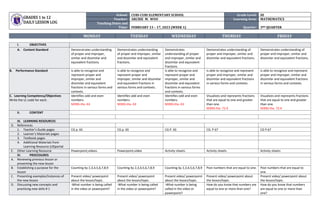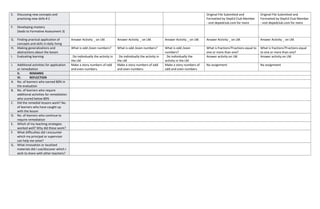# DLL_MATHEMATICS 3_Q3_W1.docx

20 Feb 20231 sur 2

### DLL_MATHEMATICS 3_Q3_W1.docx

• 1. GRADES 1 to 12 DAILY LESSON LOG School: CUBI-CUBI ELEMENTARY SCHOOL Grade Level: III Teacher: ARCHIE M. WOO Learning Area: MATHEMATICS Teaching Dates and Time: FEBRUARY 13 – 17, 2023 (WEEK 1) Quarter: 3RD QUARTER MONDAY TUESDAY WEDNESDAY THURDAY FRIDAY I. OBJECTIVES A. Content Standard Demonstrates understanding of proper and improper, similar and dissimilar and equivalent fractions. Demonstrates understanding of proper and improper, similar and dissimilar and equivalent fractions. Demonstrates understanding of proper and improper, similar and dissimilar and equivalent fractions. Demonstrates understanding of proper and improper, similar and dissimilar and equivalent fractions. Demonstrates understanding of proper and improper, similar and dissimilar and equivalent fractions. B. Performance Standard Is able to recognize and represent proper and improper, similar and dissimilar and equivalent fractions in various forms and contexts. Is able to recognize and represent proper and improper, similar and dissimilar and equivalent fractions in various forms and contexts. Is able to recognize and represent proper and improper, similar and dissimilar and equivalent fractions in various forms and contexts. Is able to recognize and represent proper and improper, similar and dissimilar and equivalent fractions in various forms and contexts. Is able to recognize and represent proper and improper, similar and dissimilar and equivalent fractions in various forms and contexts. C. Learning Competency/Objectives Write the LC code for each. Identifies odd and even numbers. M3NS-IIIa- 63 Identifies odd and even numbers. M3NS-IIIa- 63 Identifies odd and even numbers. M3NS-IIIa- 63 Visualizes and represents fractions that are equal to one and greater than one. M3NS-IIIa- 72.4 Visualizes and represents fractions that are equal to one and greater than one. M3NS-IIIa- 72.4 II. CONTENT . III. LEARNING RESOURCES D. References 1. Teacher’s Guide pages CG p. 65 CG p. 65 CG P. 65 CG. P 67 CG P.67 2. Learner’s Materials pages 3. Textbook pages 4. Additional Materials from Learning Resource (LR)portal E. Other Learning Resource Powerpoint,videos Powerpoint,video Activity sheets Activity sheets Activity sheets IV. PROCEDURES A. Reviewing previous lesson or presenting the new lesson B. Establishing a purpose for the lesson Counting by 2,3,4,5,6,7,8,9 Counting by 2,3,4,5,6,7,8,9 Counting by 2,3,4,5,6,7,8,9 Post numbers that are equal to one. Post numbers that are equal to one. C. Presenting examples/Instances of the new lesson Present video/ powerpoint about the lesson/topic. Present video/ powerpoint about the lesson/topic. Present video/ powerpoint about the lesson/topic. Present video/ powerpoint about the lesson/topic. Present video/ powerpoint about the lesson/topic. D. Discussing new concepts and practicing new skills # 1 -What number is being called in the video or powerpoint? -What number is being called in the video or powerpoint? -What number is being called in the video or powerpoint? How do you know that numbers are equal to one or more than one? How do you know that numbers are equal to one or more than one?